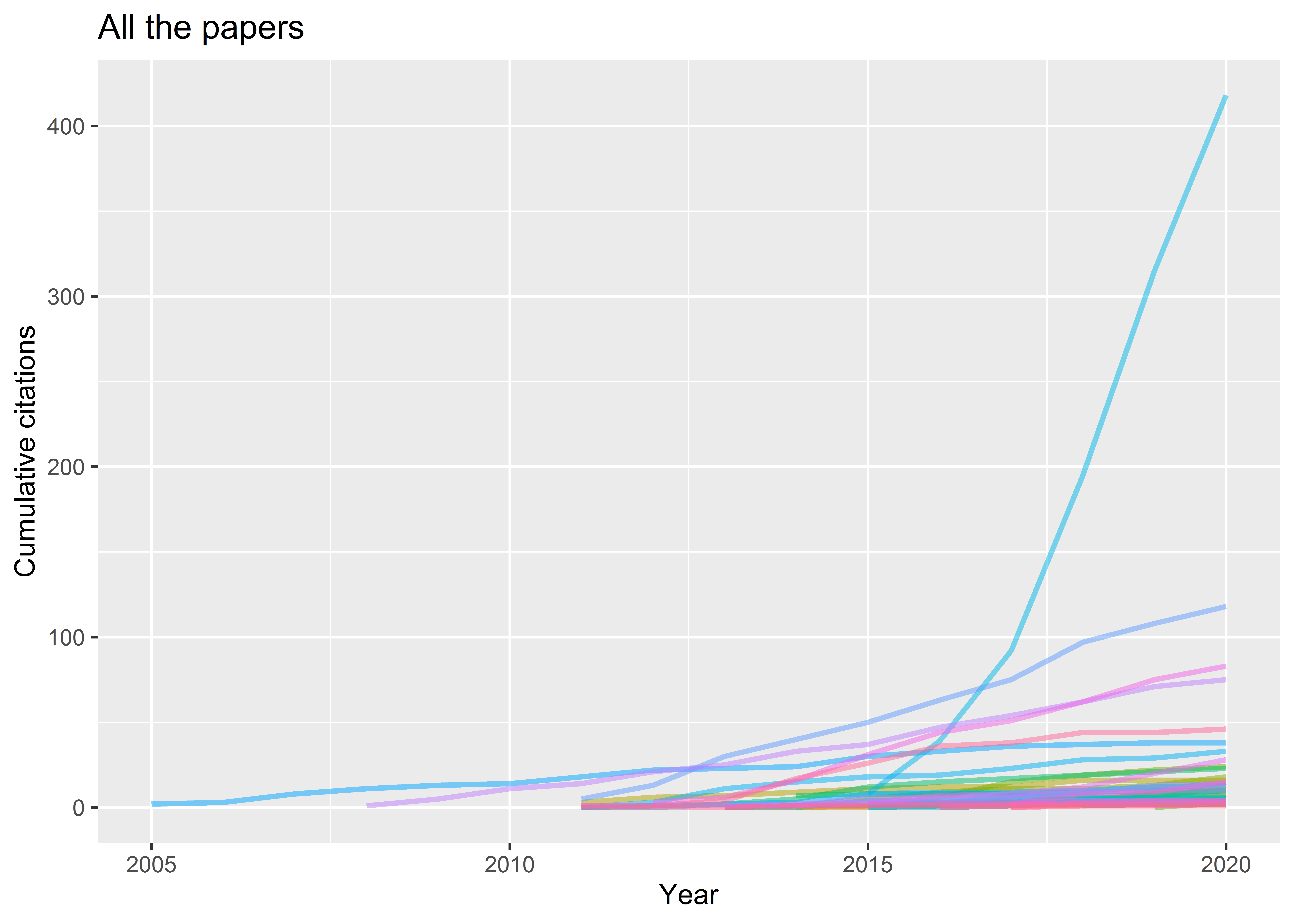This page shows how to plot citations data using the `scholar` package by Guangchuang Yu et al. and the `tidyverse`.

``````library(scholar)
library(tidyverse)``````

I’ll use my citations data:

``````andi_id <- "xrY7bFYAAAAJ"
pubs <- get_publications(andi_id)``````

Now `pubs` has a bunch of info on each of my papers:

``names(pubs)``
``````##  "title"   "author"  "journal" "number"  "cites"   "year"    "cid"
##  "pubid"``````

Here’s the current year, which will come in handy later.

``current_year <- as.numeric(format(Sys.Date(), "%Y"))``

It was 2020 when I ran this.

Now let’s look at citations each paper got by year. Get all citation data:

``````all_cites <- map_dfr(pubs\$pubid,
~ get_article_cite_history(andi_id, .x))``````

This has one row per year and original cited paper:

``head(all_cites)``
``````##   year cites        pubid
## 1 2015     8 PaBasH6fAo0C
## 2 2016    31 PaBasH6fAo0C
## 3 2017    53 PaBasH6fAo0C
## 4 2018   103 PaBasH6fAo0C
## 5 2019   120 PaBasH6fAo0C
## 6 2020   103 PaBasH6fAo0C``````

Now glue the original paper info back on. First reduce it a bit:

``````red_pubs <- pubs %>%
mutate(paper_year = year,
total_cites = cites) %>%
select(title, author, paper_year, journal, pubid, total_cites)``````

Join:

``````all_cites_pub <- all_cites %>%
left_join(red_pubs)``````
``## Joining, by = "pubid"``

Next, we need to add in implicit zero-cites where a year is missing, from the publication year up to current year.

This function does it for one paper’s cite info:

``````complete_paper_cites <- function(one_paper) {
expand_years <- setdiff(unique(one_paper\$paper_year):current_year,
one_paper\$year)
complete(one_paper,
year = expand_years,
cites = 0,
pubid,
title,
author,
paper_year,
journal,
total_cites) %>%
arrange(year)
} ``````

Give it a quick test using this input:

``````test_input <- all_cites_pub %>%
filter(pubid == "43bX7VzcjpAC")

test_input %>%
select(paper_year, year, cites)``````
``````##   paper_year year cites
## 1       2013 2016     1
## 2       2013 2017     0
## 3       2013 2018     0
## 4       2013 2019     1``````

Here’s the output:

``````complete_paper_cites(test_input) %>%
select(paper_year, year, cites)``````
``````## # A tibble: 8 x 3
##   paper_year  year cites
##        <dbl> <dbl> <dbl>
## 1       2013  2013     0
## 2       2013  2014     0
## 3       2013  2015     0
## 4       2013  2016     1
## 5       2013  2017     0
## 6       2013  2018     0
## 7       2013  2019     1
## 8       2013  2020     0``````

Looks good, so tidy up:

``rm(test_input)``

Now do this for all papers:

``````all_cites_filled <- all_cites_pub %>%
group_split(pubid) %>%
map_dfr(complete_paper_cites) %>%
mutate(age = year - paper_year)``````

Calculate the cumulative citation sums:

``````all_cites_cum <- all_cites_filled %>%
group_by(pubid) %>%
mutate(cum_sum = cumsum(cites))``````

Plot for all papers…

``````all_cites_cum %>%
ggplot(aes(x = year, y = cum_sum, colour = pubid)) +
geom_line(size = 1, alpha = 0.5) +
theme(legend.position = "none") +
labs(x = "Year",
y = "Cumulative citations",
title = "All the papers")``````That’s quite busy so try again.

``````min_cites <- 40

to_plot <- all_cites_cum %>%
filter(total_cites >= min_cites)

to_plot %>%
ggplot(aes(x = year,
y = cum_sum,
colour = reorder(str_trunc(title, 35), desc(total_cites)))) +
stat_smooth(geom="line", size = 1.2, alpha = 0.5) +
theme(legend.position = "right",
legend.directio = "vertical") +
labs(x = "Year",
y = "Cumulative citations",
colour = "Paper",
title = paste("Papers cited at least", min_cites, "times")) +
scale_x_continuous(breaks = seq(min(to_plot\$year),max(to_plot\$year)+2, 2))``````
``## `geom_smooth()` using method = 'loess' and formula 'y ~ x'``